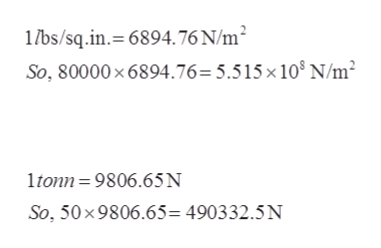# What is the cross sectional area necessary to carry a 50 ton force (tensile load) in a square bar with a tensile strength of 80000 ibs/sq in?

Question

What is the cross sectional area necessary to carry a 50 ton force (tensile load) in a square bar with a tensile strength of 80000 ibs/sq in?

check_circle

Step 1

Given:

Force F = 50 tonns

Tensile strength σ = 80,000 ibs/sq. in.

Cross-sectional area A = ?

Step 2

The formula to calculate the area of the square bar is:

Where, σ is the tensile stress, F is the force and A is the area of the square bar.

Step 3

First, convert the tensile strength in Newton/meter2....help_outlineImage Transcriptionclose1bs/sq.in. 6894.76 N/m2 So, 80000 x 6894.76=5.515 x 10° N/m ltonn 9806.65N So, 50x 9806.65= 490332.5N fullscreen

### Want to see the full answer?

See Solution

#### Want to see this answer and more?

Solutions are written by subject experts who are available 24/7. Questions are typically answered within 1 hour.*

See Solution
*Response times may vary by subject and question.
Tagged in

### Classical Mechanics